# Basic functions - math word problems

#### Number of problems found: 2267

• GranddaughterIn 2014, the sum of the ages of Meghan's aunt, her daughter and her granddaughter was equal to 100 years. In what year was the granddaughter born, if we know that the age of each can be expressed as the power of two?
• Beer permilleIn the 5 kg of blood of adult human after three 10° beers consumed shortly after another is 6.6 g of the alcohol. How much is it as per mille?
• Repairman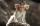Repairman has vowed to do repair work at the plant for 25 days. However work had to be shortened, and therefore he took helper worker. Together they made all the corrections for the whole days. How long it would take work to helper worker?
• TrapezoidArea of trapezoid is 135 cm2. Sides a, c and height h are in a ratio 6:4:3. How long are a,c and h? Make calculation...
• Dividing moneyVilem, Cenek, and Edita divided the money they earned by spreading the leaflet. Vilem got 240 CZK more than Cenek and twice more than Edita. Edita got 400kc less than Vilem.
• Crown coinsJana saves two-crown and five-crown coins. She has ten coins in the cashier. How many two crowns and how many five crowns shw have if she saved 29 crowns?
• Church roof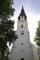The roof of the church tower has the shape of a regular tetrahedral pyramid with base edge length 5.4 meters and a height 5 m. It was found that needs to be corrected 27% covering of the roof area. What amount of material will be required?
• Jane classWhen asked how many students are in class, Jane said, if we increase the number of students in our class by hundred % and then add half the number of students, we get 100. How many students are in Jane's class?
• RatiosReduce the numbers: 50 in a 1:2 ratio 111 at a ratio of 2:3 70 at 10:50 560 at a ratio of 3:8
• Shoemaker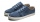Both the shoemaker and his apprentice repaired his shoes. The apprentice worked for 6 days and repaired 10 pairs of shoes every day. The shoemaker did the same job in 4 days. How many pairs of shoes did the shoemaker repair per day?
• No. 215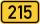From the number 215 we can create a four-digit number that among its numbers manually type any other digit. Thus, we created two four-digit numbers whose difference is 120. What two four-digit numbers that might be?
• Barrel 2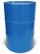Empty barrel weighs 7 kg. When it filled with water up to 45% height, weighs 60 kg. How heavy is barrel full of water?
• Ships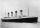At 8:40 the ship set sail at 12 km/h. At 19:10 followed by at 29 km/h sail boat. When sail boat catches up the ship? How many minutes will catch up took?
• Olive seeds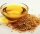5 kg of oil is pressed from 100 kg of seeds. How many kgs of seeds do we need to produce 85 kg of oil?
• Nine balls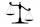Imagine that you have exactly the same appearance nine balls of which one has a greater mass than the other. You have isosceles weights. Post a procedure as you would using a weights to discover heavier balls. How many measurements at least you have to do
• Vinegar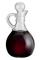We must dilute 16 liters of 8.8% aqueous vinegar to 4.1% one. How much water is necessary to add?
• The manufacturer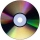The manufacturer found that 3% of the plates produced had a malfunction. Of the compliant, 75% are first and 25% second. What is the probability of producing first and second class plates?
• CoinsDenis and Zdeno together have 97 coins. If Denis had 4 coins less than he has now, the number of the coins would be in the ratio 14: 17. Determine the number of coins owned by Denis and Zdeno.
• Cheetah vs antelope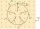When the cheetah began chasing the antelope, the distance between them was 120 meters. Although the antelope was running at 72km/h, the cheetah caught up with it in 12 seconds. What speed was the cheetah running?
• Sputnik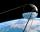The first Earth satellite was flying at speed 8000 m/s. At that rate he circled the earth in 82 minutes. Jet flies at an average speed 800 km/h. How long would it take circle the earth round?

Do you have an interesting mathematical word problem that you can't solve it? Submit a math problem, and we can try to solve it.

We will send a solution to your e-mail address. Solved examples are also published here. Please enter the e-mail correctly and check whether you don't have a full mailbox.

Please do not submit problems from current active competitions such as Mathematical Olympiad, correspondence seminars etc...